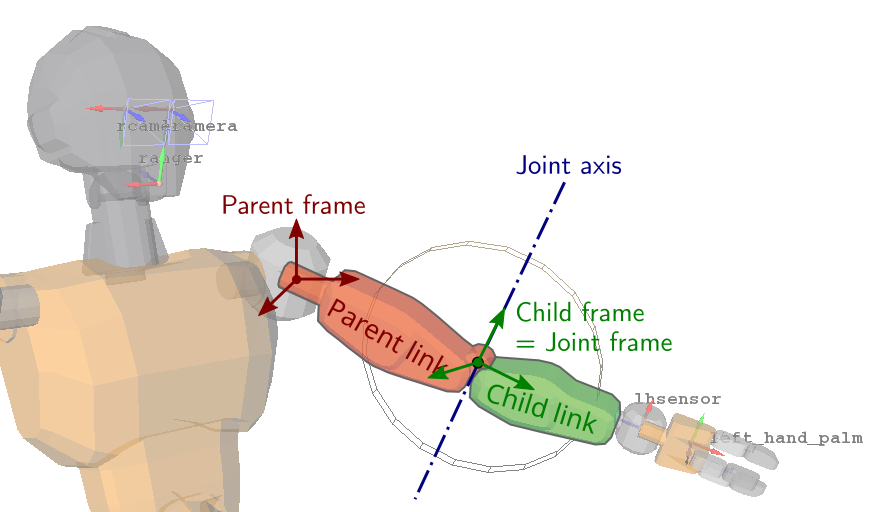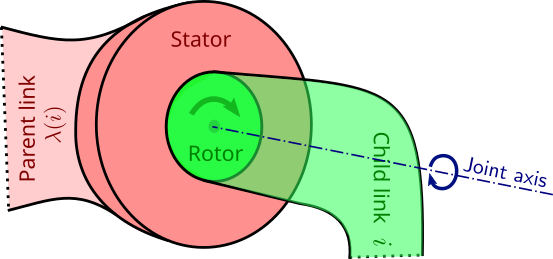# Revolute joints

Revolute joints are the most common ones in legged robots. Their equations of motion involve both joint coordinates $$\bfq$$ and joint torques $$\bftau$$:

\begin{equation*} \bfM(\bfq) \qdd + \qd^\top \bfC(\bfq) \qd = \bfS^\top \bftau + \bftau_g(\bfq) + \bfJ_c(\bfq)^\top \bff \end{equation*}

But given a joint $$i$$ connecting a link $$i$$ to its parent $$\lambda(i)$$, what is the definition of the joint angle $$q_i$$ in $$\bfq$$? Or of the joint torque $$\tau_i$$ in $$\bftau$$?

## Kinematics of a revolute joint

Our robot model typically consists in a kinematic tree rooted at a special link, the floating base or base link. Each link is a rigid body connected to one or more others by joints that prevent motion in some axes while allowing it on some others. Here is the example of a revolute joint at the elbow of a humanoid robot:In general there are six degrees of freedom between two rigid bodies, corresponding to the three translation and three rotation coordinates of their relative pose. A revolute joint allows rotation around one axis only, the joint axis, thus leaving one degree of freedom while applying five degrees of constraint between the two bodies. Such a joint is realized in practice by a rotary actuator, which looks like this:Regardless of its design (brushless DC electric, series-elastic, quasi-direct drive, …), the rotary actuator always has two main bodies when we look at it from the outside: a stator and a rotor. The rotor rotates around (and is typically symmetric around) the joint axis. The stator is attached to one link and the rotor to the other, so that the actuator applies joint torque between the two links. In the figure above we have attached the stator to the parent link and the rotor to the child link, but it could very well have been the other way round.

## Dynamics of a revolute joint

While kinematics derives from the composition of transforms $$\bfX_{CA} = \bfX_{CB} \bfX_{BA}$$, dynamics derives from the Newton-Euler equations of rigid bodies:

\begin{equation*} \bfI_i \bfa_i + \bfv_i \times^* \bfI_i \bfv_i = \bfw^{net}_i \end{equation*}

where $$\bfa_i$$ is the spatial acceleration of the link, $$\bfI_i$$ its spatial inertia matrix, and $$\bfw^{net}_i$$ the net spatial force applied to it. The left-hand side represents the inertia and motion of the link, while the right-hand side represents forces. Let us focus on the latter to see how the joint torque $$\tau_i$$ appears there.

## To go further

You can take a bite at the knee torque of a lumped mass problem to check whether you are at ease with the kinematics and dynamics of revolute joints. The formulas we have seen here are presented for other types of joints in Roy Featherstone's Rigid Body Dynamics Algorithms. Check out in particular sections 3.5 (Joint Constraints) and 4.4 (Joint Models).

## Discussion

You can use Markdown with $\LaTeX$ formulas in your comment.